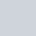# Operators & Control structureWritten by

In this post i try to explain you javascript operators and control structure . Operators are very helpful for programmer , because it helps to programmer to solve arithmetic& logical problems like addition, subtraction, division etc. and also control structure help to control the flow of program.# Operator

JavaScript has the following types of operators.

• Assignment operators
• Comparison operators
• Arithmetic operators
• Bitwise operators
• Logical operators

## Assignment operators

Assignment operator use when you assign and value in any variable and then you want to change your variable value by adding any other variable or multiplying to any other variable etc.,

TYPEMEANING
`x += y``x = x + y                 `
`x -= y``x = x - y`
`x *= y``x = x * y`
`x /= y``x = x / y`
`x %= y``x = x % y`

## Comparison Operator

Comparison operator use for compare two values and it return true value if the condition is true  and also these operator use for conditional statement .

OPERATORDESCRIPTION
Equal (`==`)Returns true if the both value or variable value are equal.
Not equal (`!=`)Returns true if the both value or variable value are not equal.
Strict equal (`===`)Returns true if the both value or variable value are equal and of the same type.
Strict not equal (`!==`)Returns true if the both value or variable value are not equal and/or not of the same type.
Greater than (`>`)Returns true if the left value or variable value is greater than the right operand.
Greater than or equal (`>=`)Returns true if the left value or variable value is greater than or equal to the right operand.
Less than (`<`)Returns true if the left value or variable value   is less than the right operand.
Less than or equal (`<=`)Returns true if the left value or variable value  is less than or equal to the right operand.

## Arithmetic Operator

Arithmetic operators use for solve arithmetic question like add two value and return a single numerical value. Basically very common arithmetic operator is + for addition – for subtraction . All the arithmetic operators are shown in below table .

OPERATORDESCRIPTION
`%`
(Modulus)
use for modulus and it return integer value means if the 5%4 = 1
`++`
(Increment)
unary operator use for increment the value 1 in value.
`--`
(Decrement)
Unary operator. Subtracts one from variable value.

## Logical operators

OPERATORDESCRIPTION
`&&`(Logical AND) it returns true if the both condition or values are same.
`||`(Logical OR) Returns true if the any one condition or value is true.
`!`it return true if the condition is false or value is false.

## Control Structure

control structure help to programmer to control the program . In control structure we apply some condition and we check this by the help of if  else statement Like below

```var a=prompt("Enter number!");
if(a !==2)
{
console.log("a is not equal to two");
}
else
{
console.log("a is equal to two");
}```

Prompt will give popup box and will take entry of number and store in variable “a”. Like this

Syntax of if else statement

if (condition) {
statement 1 runs if condition is true} else {
statement 2 runs if condition is false}

## Switch statement

switch statement is very cool statement . Basically we have used switch statement if the lots of possible condition are available like book type etc.

## Basic Syntax

switch (expression) {
case label_1:
statements_1
break;
case label_2:
statements_2
break;
default:
statements_def
break;
}

Example

```var name=prompt("Enter your name");
switch (name) {
case "sam":
console.log("hi i am "+name);
break;
case "ak":
console.log("hi i am "+name);
break;

default:
break;
}```

in:

Tagged:

Previous article

Variables & Data types

Next article

Functions & Predefined Functions

## Join the discussion

This site uses Akismet to reduce spam. Learn how your comment data is processed.